MORE SITES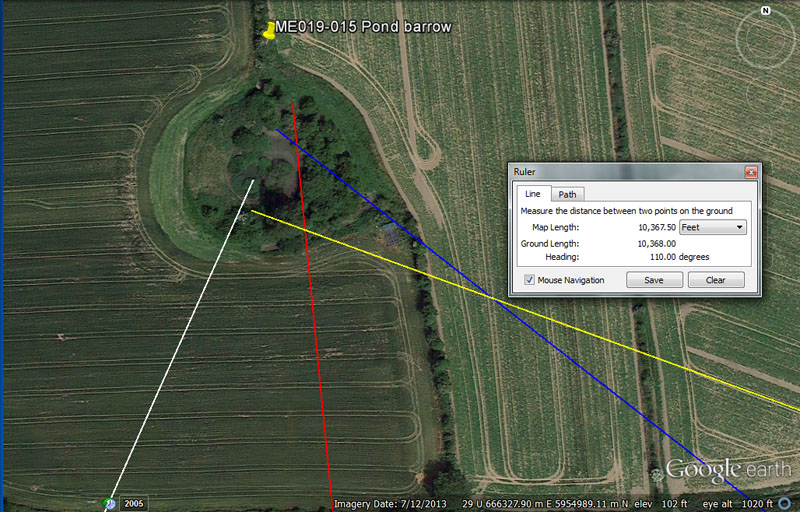Building this large pond barrow must have been an ambitious undertaking for the ancient engineers providing artificial island classrooms for the sprawling open-air-university. The dynamic codes extractible in this position attest to its importance.

From  Knowth = 6300’ (also 6336’) @ 24-degrees.

A distance of 6300' is 10 stadia or 1.2-miles of 5250'. It is also the centre to centre distance from the Octagon of Newark, Ohio USA to a nearby earth embankment structure called the Great Circle. Alternatively 6336' would be 1.2-miles of 5280'.

The degree angle (1/15th of 360-degrees) is self explanatory.

From Newgrange = 8250’ @ 354.375-degrees.

This distance is half a league.

The degree angle relates to the lunar year of 354.375-days.

From Dowth Mound = 7920’ @ 128-degrees return.

This distance is 1.5-miles of 5280' and is in homage to the diameter of the Earth @ 7920-miles.

The degree angle is reminiscent of dividing up the compass into 32-divisions then subdividing yet further to 128-divisions.

From Dowth Henge = 10368 @ 110- degrees return.

The distance code related to the rate of rotation of the Earth per day @ 1036.8 MPH.

The angle encodes the "11" series of navigational numbers and 110' would be 1/48th of a 5280'-mile.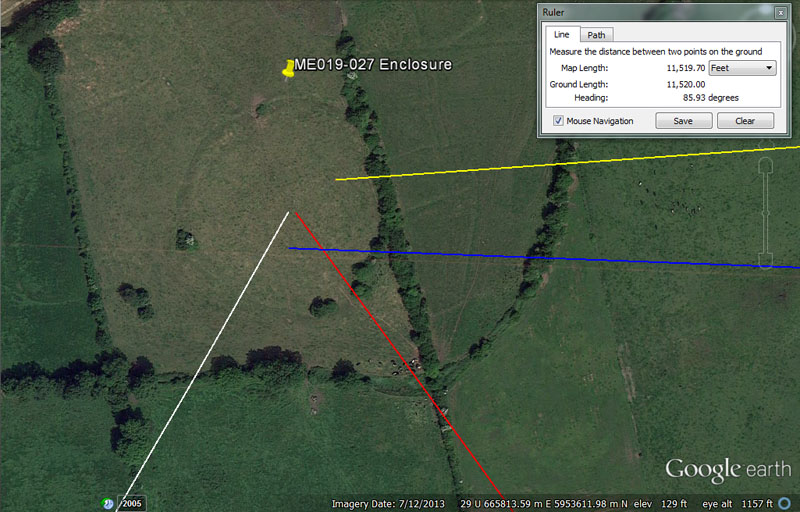This is a large enclosure near to Knowth chambered mound.

From Knowth = 1512 @ 29.7-degrees.

Again, in this length is another expression, in reduced scale, to the 25-minutes of equatorial arc between the centre of Dowth Henge and the summit chambered cairn atop Slieve Gullion Mountain in County Armagh. By consequence, this distance is .25 of 1-minute of arc.

The degree angle related to the 5940' Scottish mile, of which 2970' = half.

From Newgrange = 4536’ @ 144-degrees.

The sum of 4536' would be 1/28800th of the equatorial circumference under the 24883.2-mile (of 5250') navigational method. Also, nowhere in recorded history has there been mention of a pointed capstone observed atop the Great Pyramid of Egypt and the coded-mathematical, as well as measurable, evidence shows that it had a top floor altar at the vertical height of 453.6'. This would be 264 Egyptian Royal Cubits of 20.618".

The 4536' encoding in the Boyne River Valley of Ireland could be equally read as 4537.5 to accommodate the 20.625" Egyptian Royal Cubit.

The degree angle accentuates the Geomancer's mile (14400') or the Egyptian Pyramid acre (28800 square feet), etc., and in a mathematical progression generates many lunar-cycle, precession, calendar and navigational values.

From Dowth Mound 8100 @ 92.16 – 272.16-degrees.

This distance is in homage to rounded PHI (1.62) and 8100' is half of 16200'. The length of 8100' is 1 & 2/3rds so-called Roman miles of 4860'.

The degree angle is dynamic coding in both directions. The sum of 69.12-miles would of course, be 1-degree of arc for a world of 24883.2-miles. At the same time, 27216' would be 1/4800th of the 24883.2-mile circumference (130636800').

From Dowth Henge = 11520 @ 85.9375-degrees.

This length is navigational coding and 115.2-miles would equate to 1/216th of the 24883.2 circumference.

The degree angle is in homage to 1/8th of 1-degree of equatorial arc. Under the 69.12-mile (of 5250') method that would equate to 45360'. Alternatively, under the 68.75-mile (of 5280') method that would equate to 45375'. The sum of 68.75-miles ÷ 8 = 8.59375-miles (8 & 19/32nds).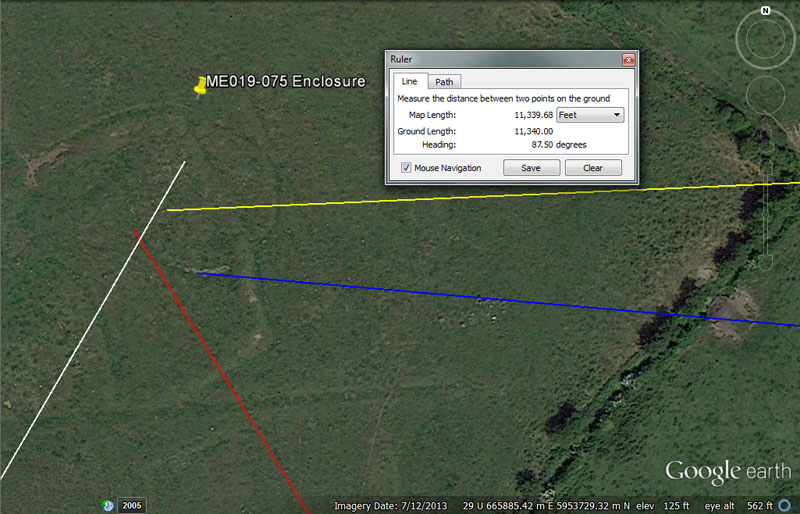Yet another enclosure sits nearby.

From Knowth = 1980’ @ 30 - 210-degrees. This would also have complied to 1968.75’ @ 30.24-degrees.

This distance is 1/3rd of an ancient Scottish mile of 5940' & 3/8ths of a 5280'-mile. Also, 1980-miles of 5280' would be 1/4th of the diameter of the Earth (7920-miles).

Within the confines of the site a distance of 1968.75' was also achievable and this lunar-related value was a very important to ancient assayers. The sum of 19.6875-days (19 & 11/16ths) would be 1/18th of a lunar year of 354.375-days. A distance of 1.5-miles of 5250' (7875') would equate to 1968.75' X 4. The ancient Egyptians, as well as the latecomer Greeks and Romans adopted the value of 196.875-grains for use in their gold standard. This was known as the Beqa weight for gold by the Egyptians & later Romans and as the Didrachma or Tridrachme by the Greeks. This standard survived amongst assayers into more modern times. The Roman Uncia weight of 393.75-grains was two Egyptian Beqa and would have been very useful to the Roman armies, who were forever plundering everyone else's gold.

The outwards running and return angles of 30 - 210-degrees are self explanatory, but the angle of 30.24-degrees was also achievable to code half a minute of equatorial arc (3024').

From Newgrange = 4725’ @ 148.5-degrees.

This distance is .9 of the 5250' mile. In the 3,4,5 triangle formula used to build the Khafre Pyramid of Egypt the height of the edifice was 472.5'. The sum of 472.5-days would be 16 lunar months of 29.53125-days each (29 & 17/32nds).

The angle of 148.5-degrees is navigational coding and 1485' would be 1/4th of the 5940'-mile.

From Dowth Mound = 7875’ @ 95.04-degrees.

This distance is 1.5 miles of 5250'.

The degree angle is also navigational coding under the 24750-mile (of 5280') equatorial circumference (130680000') and the sum of 95040' (18-miles) would be 1/1375th part.

From Dowth Henge = 11340’ @ 87.5-degrees.

This distance value is much used in lunar cycle computations and the 6804-day lunar nutation cycle is 6 intervals of 1134-days. Also, there would be 2.25 periods of 1134-days in the 2551.5-days of the lunisolar calendar. Greek historian, Herodotus was told by Egyptian priests that the Great Pyramid was 800' long. The priests were referring to an increment of 11.34" for lunar readings. Also, 3 side-lengths of the Great Pyramid equal 2268' (756' X 3) which equates to 1134' X 2.

This distance is 1 & 2/3rds miles of 5250'. The sum of 8.75-days represented the shortfall in time at the termination of the Sabbatical Calendar count (7th or Sabbatical year) and 8.75 intercalary days were added to correct the calendar for the next cycle.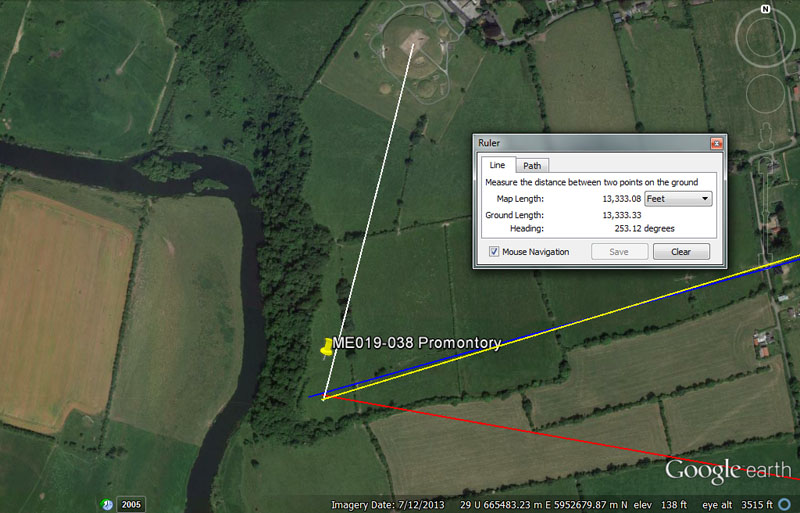There was a promontory of high vantage point here that is listed as an archaeological site.

From Knowth = 1760’ @ 194.4-degrees. Also 1771.875’.

For orienteers venturing out from Knowth chambered mound, this objective lay 1/3rd of a mile away (1760'). Similarly, they would have found the site using a distance code of 1771.875'. The lunar year @ 354.375-days is 171.1875-days ( 171 & 3/16ths) X 2.

The degree angle is navigational and 194.4-miles would be 1/128th of the 24883.2-mile equatorial circumference.

From Newgrange = 3937.5’ @ 280 - 100-degrees.

This distance is .75 of the 5250' mile. Also, the duration of 39.375-days would be 1/9th of the 354.375-day lunar year. The late era Uncia weight of the Romans was 393.75-grains.

The degree angle is self explanatory.

From Dowth Mound = 9720’ @ 74.25-degrees.

This distance is 2 so-called Roman miles.

The degree angle is navigational and the sum of 742.5' would be 1/8th of the 5940-mile.

From Dowth Henge = 13333.333333 @ 253.125.

This distance is 1/3rd of 40000'. Orienteers could also have located this site if assigned to travel 2.75 so-called Roman miles (13365').

The 253.125-degree angle is lunar coding also and there would be 14 periods of 25.3125-days (25 & 5/16ths) in a lunar year.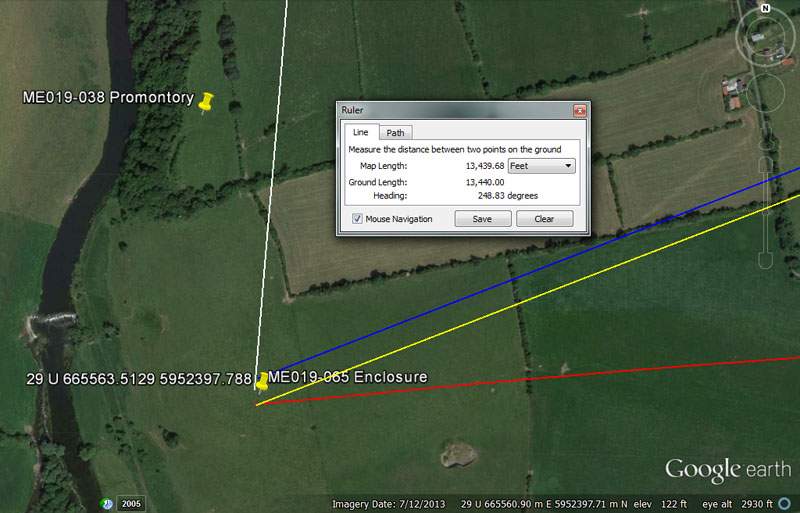Yet another enclosure.

From Knowth = 2625’ @ 5-degrees.

This distance is half a mile of 5250'.

The degree angle is self-explanatory.

From Newgrange = 3675’ @ 85.05-degrees.

This distance is .7 of the 5250'-mile.

The degree angle is lunar and the sum of 850.5-days would be 1/8th of the 6804-day lunar nutation cycle.

From Dowth = 9720’ @ 68.75-degrees.

This distance is two so-called Roman miles of 4860'.

The degree angle is in homage to the 68.75-miles in 1-degree of equatorial arc under the 24750-mile navigational method.

From Dowth Henge = 13440’ @ 248.832-degrees.

The distance of 13440' would equate to 2 ancient Irish miles of 6720'.

The degree angle encodes the 24883.2-mile equatorial circumference.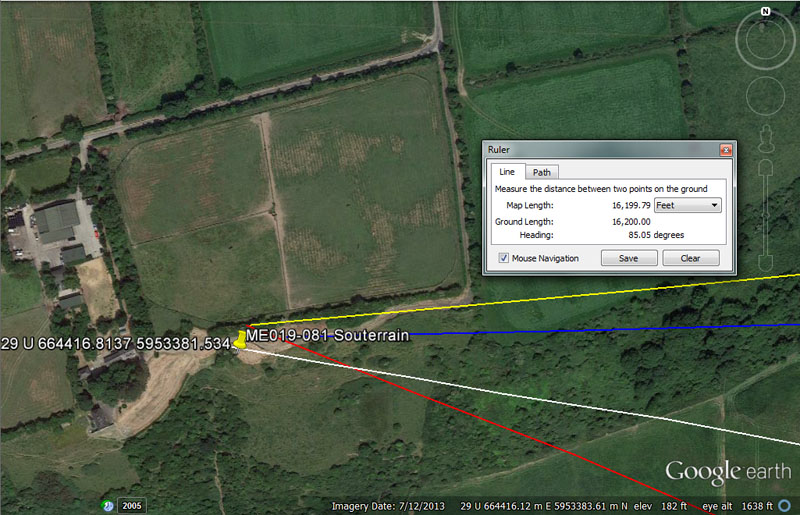Orienteers could find a large souterrain complex here at Crewbane atop an escarpment and overlooking the Boyne River. Adjacent was an enclosure and settlement.

From Knowth = 3937.5’ @ 100.8-degrees.

This distance is .75 of the 5250' mile. Also, the duration of 39.375-days would be 1/9th of the 354.375-day lunar year. The late era Uncia weight of the Romans was 393.75-grains.

The degree angle is in homage to the 100.8' in 1-second of equatorial circumference arc for the world.

From Newgrange = 7920’ @ 113.4-degrees.

This distance is 1.5-miles of 5280' and also encodes the diameter of the Earth @ 7920-miles.

This angle encodes the lunar number 1134 and there would be 6 intervals of 1134-days in the lunar nutation cycle of 6804-days, etc.

From Dowth Mound = 12600’ @ 89.1-degrees.

This distance would be 2.4-miles of 5250' or 1200 reeds of 10.5'. It would also be 12000 Greek feet, 20 stadia or 1/12th of the 151200' (25-minutes of arc) distance between the centre of Dowth Henge and the chambered trig cairn atop Slieve Gullion Mountain in County Armagh.

The angle relates to the 5940' mile, for which 8910' would equate to 1.5 such miles.

From Dowth Henge = 16200’ @ 85.05-degrees return.

The distance encodes readings in rounded PHI increments based upon 1.62 to 1. A distance of 16200' equates to 3 & 1/3rd so-called Roman miles. The value 162 was used copiously in all manner of navigational and lunar computations.

The degree angle reading relates to an incremental value within the 6804-day lunar nutation cycle of which 85.05-days would be 1/80th part.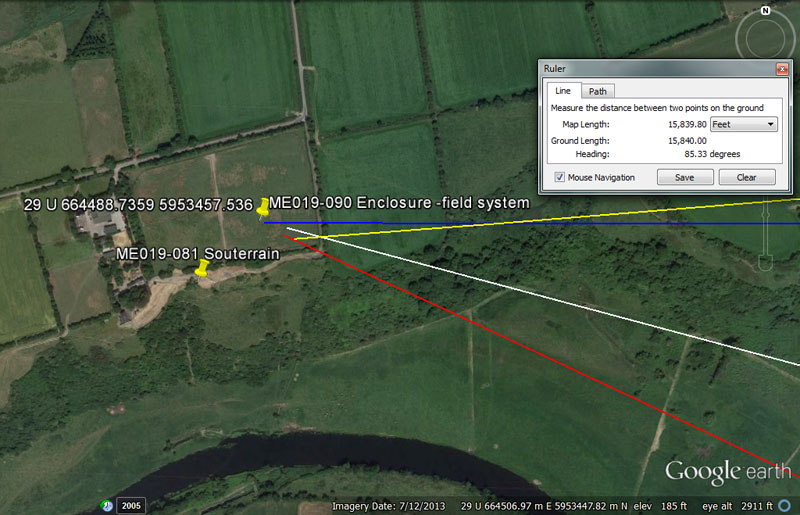Near the Crewbane souterrain accommodation was an enclosure and settlement where, undoubtedly, the students could receive instruction and sustenance.

From Knowth = 3628.8’ @ 105-degrees.

The distance encodes the value for 1-degree of arc or 60-minutes of equatorial arc, which was 362880'. This distance to the Crewbane site is .6 of 1-minute of arc.

The angle encodes the values 10.5' (1-reed) or 10500' (2-miles of 5250' each).

From Newgrange = 7680’ @ 115.2.

The distance of 7680' would be 1/17010st of the 130636800' equatorial circumference. Of course, 1701-days would be 1/4th of the lunar nutation cycle. In the 69.12-mile reading for 1-degree of equatorial arc. the sum of 7.68-miles would be 1/9th part.

The degree angle is navigational also and 115.2-miles would equate to 1/216th of the 24883.2 circumference.

From Dowth Mound = 12441.6’ @ 90-degrees.

This distance encodes the 24883.2-mile equatorial circumference, of which 12441.6-miles would be the half value.

The degree angle would represent due East, where the equinoctial sun rises.

From Dowth Henge = 15840’ @ 85.33333-degrees.

This distance is 3-miles of 5280' each.

The angle provides a value that is 1/3rd of 256. It would be in homage to dividing up the 360-degree circle into divisions of 32 and then further subdivision refinements.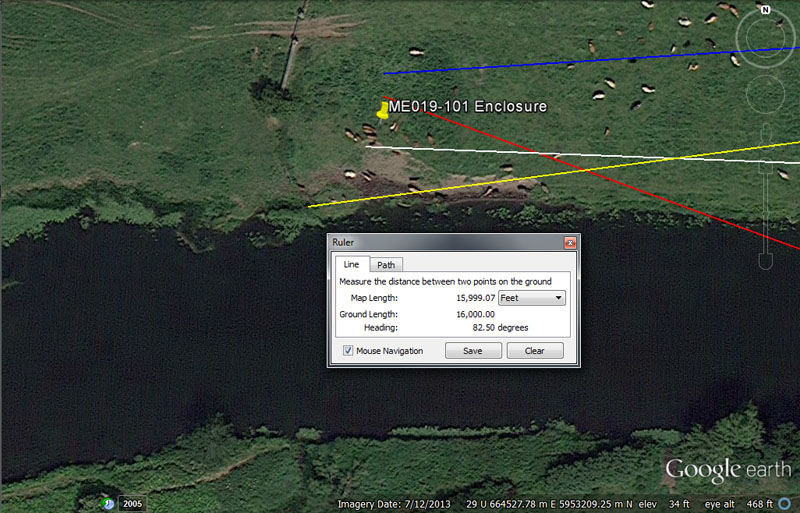Below the escarpment at Crewbane, on the river bank, is found the clear remnants of a formerly impressive enclosure.

From Knowth = 3520’ @ 93.3120-degrees.

This distance is 2/3rds of a mile of 5280'.

The degree angle encodes a much used value of antiquity that found its way into the weights and measures standards of several civilisations. For example, the Sumerian / Babylonian heavy Talent was 933120-grains. The sum of 933120' would be 1/140th of the 24883.2-mile (130636800') equatorial circumference of the Earth.

From Newgrange = 7382.8125’ @ 110-degrees.

The distance code 7382.8125 (7382 & 13/16ths) is lunar and, in days, this value would equate to 250 lunar months.

The degree angle is self-explanatory.

From Dowth Mound = 12375’ @ 86.4-degrees.

This length, in miles, would be half of the 24750-mile equatorial circumference. Rendered as 12375' it would also equate to 7200 Egyptian Royal cubits of 20.625", 2250 merchant fathoms, 750 rods or perches, 187.5 chains, 18.75 furlongs or furrowlongs and is .75 of a league.

The angle code accentuates a very important value to all-manner of ancient calculations. The sum of 8640-years would be 1/3rd of the 25920-year Precession of the equinoxes cycle and 8640' would be 1/7th of 10-minutes of equatorial arc (60480'). The sun is 864,000-miles wide.

From Dowth Henge = 16000’ @ 262.5-degrees.

The distance is self-explanatory.

The degree angle relates to the 5250'-mile of which 2625' is the half value.